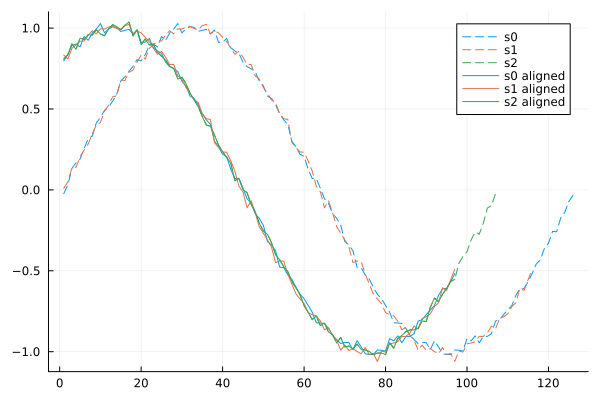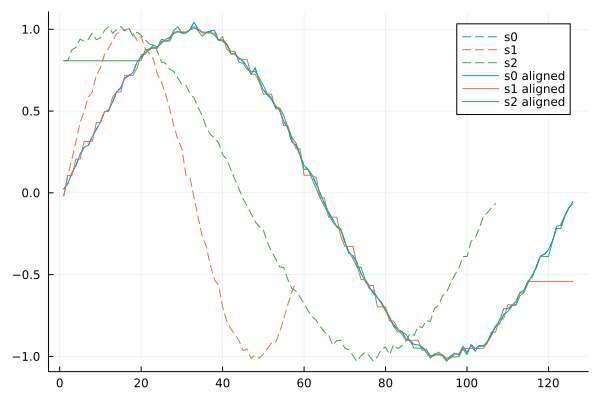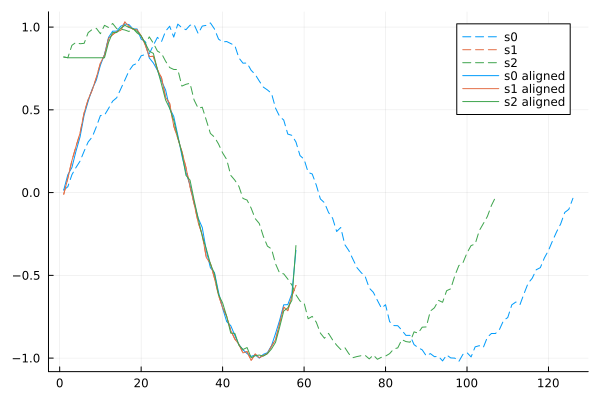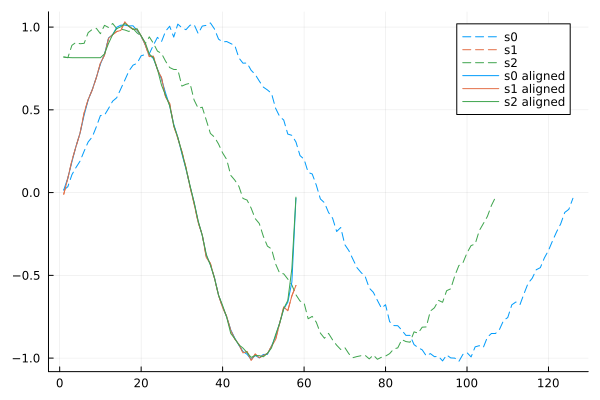## SignalAlignment.jl

Align signals to each other
Author baggepinnen
Popularity
9 Stars
Updated Last
5 Months Ago
Started In
January 2021

# SignalAlignment

This package takes a vector of signals and tries to align them.

One use case for this is when two different instruments are used to record something that is going on, but they may record at different sample rates, have no synchronization, or have different time offsets. Before analyzing such experiments, it may be helpful to align the signals to each other.

# Function reference

• `align_signals(signals, method; master, by, output)`: Main entrypoint for signal alignment
• `syncplot`: takes the same arguments as `align_signals` (except `output`) and plots the aligned signals

## Method reference

The method indicates how alignment is computed. The method is specified by passing a `method` argument to `align_signals`. The following methods are available:

• `Delay(; delay_method)`: Align signals by shifting them with respect to each other
• `delay_method = DTWDelay()`: Align signals by computing the optimal delay using Dynamic-Time Warping. This can be computationally expensive for very long signals, but is more robust than `XcorrDelay`.
• `delay_method = XcorrDelay()`: Align signals by computing the optimal delay using cross-correlation
• `Warp(; warp_method)`: Align signals by warping them with respect to each other
• `warp_method = DTW(; radius, ...)`: Align signals by computing the optimal warp using Dynamic-Time Warping. See DynamicAxisWarping.jl for options to `DTW`.
• `warp_method = GDTW()`: Align signals by computing the optimal warp using Generalized Dynamic-Time Warping. See DynamicAxisWarping.jl for options to `GDTW` or the example below.

## Master reference

The master indicates which signal is used as the reference signal to which the other signals are aligned. The master is specified by passing a `master` argument to `align_signals`. The following masters are available:

• `Index`: Align all signals to a particular signal. The default is `Index(1)` which aligns all signals to the first signal.
• `Longest`: Align all signals to the longest signal
• `Shortest`: Align all signals to the shortest signal
• `Centroid`: Align all signals to the computed centroid (generalized median) of all signals. The metric used to compute the centroid is specified by, e.g., `Centroid(SqEuclidean())`.
• `Barycenter`: Align all signals to the computed barycenter (generalized mean) of all signals. The metric used to compute the barycenter is specified by, e.g., `Barycenter(SqEuclidean())`.

## Output reference

The output indicates what is returned by `align_signals`. The output is specified by passing an `output` argument to `align_signals`. The following output options are available:

• `Indices()`: Return the indices that align the signals.
• `Signals()`: Return the aligned signals.

The map from indices to aligned signals is

`aligned_signals = [signals[i][inds[i]] for i in eachindex(signals)]`

# Examples

## Align shifted signals to one particular signal

We can indicate that we want to align a vector of signals to a particular signal by passing the index of the signal we want to align to as the `master` argument to `align_signals`. The default master if none is provided is `Index(1)` like we use below.

```using SignalAlignment
s0 = sin.((0:0.05:2pi)) # A noisy signal
s1 = s0[1:end-10]       # A second signal, misaligned with the first
s2 = s0[20:end]         # A third signal
signals = [s0, s1, s2]  # A vector of signals we want to align
signals = [s .+ 0.02 .* randn(length(s)) for s in signals] # Add some noise to the signals

master = Index(1)       # Indicate which signal is the master to which the others are aligned
method = Delay(delay_method=DTWDelay()) # Indicate that we want to align the signals by shifting them, and the delay between them is computed using DTW
output = Indices() # Indicate that we want the aligning indices as output
inds = align_signals(signals, method; master, output)```
``````3-element Vector{UnitRange{Int64}}:
2:1884
2:1884
1:1883
``````

The indices returned by `align_signals` can be used to align the signals to the master signal.

```aligned_signals = [signals[i][inds[i]] for i in eachindex(signals)]
plot(signals, label=["s0" "s1" "s2"], l=(:dash, ))
plot!(aligned_signals, label=["s0 aligned" "s1 aligned" "s2 aligned"], c=(1:3)', size=(600, 400))```The example above used Dynamic-Time Warping (DTW) to find the optimal delay with which to shift the signals to the master. Rather than DTW, we can compute the delay using cross-correlation as well

`method = Delay(delay_method = XcorrDelay())`

If we want to obtain the aligned signals directly as output rather than the aligning indices, we pass `output = Signals()`.

## Align signals with different sample rates

In this example, the second signal has a sample rate that is 2x lower than the first signal. We can align the signals by warping them using Dynamic-Time Warping (DTW) to fit the first signal. DTW is handled by the DynamicAxisWarping.jl package.

```using SignalAlignment
s0 = sin.((0:0.05:2pi)) # A noisy signal
s1 = s0[1:2:end-10]     # A second signal with 2x lower sample rate
s2 = s0[20:end]         # A third signal
signals = [s0, s1, s2]  # A vector of signals we want to align
signals = [s .+ 0.02 .* randn(length(s)) for s in signals] # Add some noise to the signals

master = Index(1)       # Indicate which signal is the master to which the others are aligned
output = Signals() # Indicate that we want the aligned signals as output
# syncplot(signals, method; master) # Call this if you only want to plot the aligned signals

aligned_signals = align_signals(signals, method; master, output)
plot(signals, label=["s0" "s1" "s2"], l=(:dash, ))
plot!(aligned_signals, label=["s0 aligned" "s1 aligned" "s2 aligned"], c=(1:3)', size=(600, 400))```Notice how the signal that was sampled slowly has been stretched to fit the first signal. This introduces some artifacts, where some samples have been repeated. If undesired, these artifacts can be mitigated somewhat by using generalized DTW, shown below. If the signals are instead aligned to the shortest signal, the longer signals are subsampled:

```master = Shortest()
aligned_signals = align_signals(signals, method; master, output)
plot(signals, label=["s0" "s1" "s2"], l=(:dash, ))
plot!(aligned_signals, label=["s0 aligned" "s1 aligned" "s2 aligned"], c=(1:3)', size=(600, 400))```To get a smoother result, use generalized DTW (GDTW) instead of DTW.

```master = Shortest()
method = Warp(warp_method=GDTW(symmetric=false))
aligned_signals = align_signals(signals, method; master, output)
plot(signals, label=["s0" "s1" "s2"], l=(:dash, ))
plot!(aligned_signals, label=["s0 aligned" "s1 aligned" "s2 aligned"], c=(1:3)', size=(600, 400))```### Required Packages

View all packages

### Used By Packages

No packages found.